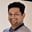Related Tags

java
community creator

# How to pass variable arguments in JavaTarun Telang

## Methods

A method is a block of code that can be called when needed. It is defined with the name of the method followed by parentheses (), and it enables code reuse as it can be defined once and run multiple times.

You can pass data, known as arguments, into a method:


public class MyClass {

public static void myMethod() {
System.out.println("Hello World");
}

public static void main(String[] args) {
myMethod(); // prints "Hello World"
}
}
example code to demonstrate method call in Java
• static means the method can be called without instantiating the object of the class.
• void means that this method does not return a value.

## Variable arguments

A variable argument is prefixed with ....

Syntax:

<returnType> <methodName>(<type> <arg>,<type> ...<vararg>)

• The Variable Argument should be the last parameter of the method.
• The Variable Argument can be used to pass zero or more arguments to the method.
public class Calculator {
public int sum(int...numbers) {
int total = 0;
for(int i: numbers) {
total += i;
}
}

public static void main(String[] args) {
Calculator calculator = new Calculator();
int number = calculator.sum(1, 3, 4, 5);
System.out.println(number); // prints 13
number = calculator.sum(2, 6);
System.out.println(number); // prints 8
}
}

RELATED TAGS

java
community creator

CONTRIBUTORTarun Telang
RELATED COURSES

View all Courses

Keep Exploring

Learn in-demand tech skills in half the time